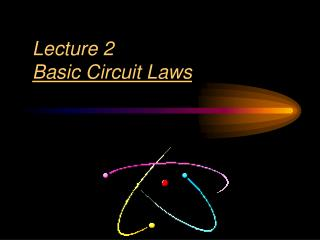DownloadDownload PresentationLecture 2 Basic Circuit Laws

# Lecture 2 Basic Circuit Laws

Télécharger la présentation## Lecture 2 Basic Circuit Laws

- - - - - - - - - - - - - - - - - - - - - - - - - - - E N D - - - - - - - - - - - - - - - - - - - - - - - - - - -
##### Presentation Transcript

1. Lecture 2Basic Circuit Laws

2. Basic Circuit Laws 2.1 Ohm’s Law. 2.2 Nodes, Branches, and Loops. 2.3 Kirchhoff’s Laws. 2.4 Series Resistors and Voltage Division. 2.5 Parallel Resistors and Current Division. 2.6 Wye-Delta Transformations.

3. 2.1 Ohms Law (1) • Ohm’s law states that the voltage across a resistor V(v)is directly proportional to the current I(A)flowing through the resistor. • When R=0 short circuit • When R=open circuit. a b

4. 2.1 Ohms Law (2) • Conductanceis the ability of an element to conduct electric current; it is the reciprocal of resistance R and is measured in siemens. • The power dissipated by a resistor:

5. 2.4 Series Resistors and Voltage Division (1) • Series: Two or more elements are in series if they are cascaded or connected sequentially and consequently carry the same current. • The equivalent resistance of any number of resistors connected in a series is the sum of the individual resistances. • The voltage divider can be expressed as

6. 2.4 Series Resistors and Voltage Division (1) As the current goes through the circuit, the charges must USE ENERGY to get through the resistor. So each individual resistor will get its own individual potential voltage). We call this voltage drop. Note: They may use the terms “effective” or “equivalent” to mean TOTAL!

7. Example R(series) = 1 + 2 + 3 = 6W A series circuit is shown to the left. • What is the total resistance? • What is the total current? • What is the current across EACH resistor? • What is the voltage drop across each resistor?( Apply Ohm's law to each resistor separately) DV=IR 12=I(6) I = 2A They EACH get 2 amps! V1W=(2)(1)= 2 V V3W=(2)(3)= 6V V2W=(2)(2)= 4V Notice that the individual VOLTAGE DROPS add up to the TOTAL!!

8. 2.5 Parallel Resistors and Current Division (1) • Parallel: Two or more elements are in parallel if they are connected to the same two nodes and consequently have the same voltage across them. • The equivalent resistance of a circuit with N resistors in parallel is: • The total current i is shared by the resistors in inverse proportion to their resistances. The current divider can be expressed as:

9. 2.5 Parallel Resistors and Current Division (1) R3 • Parallel wiring means that the devices are connected in such a way that the same voltage is applied across each device. • Multiple paths are present. • When two resistors are connected in parallel, each receives current from the battery as if the other was not present. • Therefore the two resistors connected in parallel draw more current than does either resistor alone. I3

10. Example 4 To the left is an example of a parallel circuit. a) What is the total resistance? b) What is the total current? c) What is the voltage across EACH resistor? d) What is the current drop across each resistor? (Apply Ohm's law to each resistor separately) 2.20 W 3.64 A 8 V each! Notice that the individual currents ADD to the total. 1.6 A 1.14 A 0.90 A

11. Kirchhoff's Rules Gustav Kirchhoff 1824-1887 German Physicist

12. 2.2 Nodes, Branches and Loops (1) • A branch represents a single element such as a voltage source or a resistor. • A node is the point of connection between two or more branches. • A loop is any closed path in a circuit.

13. 2.2 Nodes, Branches and Loops (2) Example 1 Original circuit Equivalent circuit How many branches, nodes and loops are there? 4 Branches 3 Nodes 4 loops

14. Should we consider it as one branch or two branches? 2.2 Nodes, Branches and Loops (3) Example 2 How many branches, nodes and loops are there? 7 Branches 4 Nodes 4 loops

15. 2.3 Kirchhoff’s Laws (1) • Kirchhoff’s current law (KCL) states that the algebraic sum of currents entering a node (or a closed boundary) is zero. Mathematically,

16. Junction Rule • Conservation of mass • Electrons entering must equal the electrons leaving • The junction rule states that the total current directed into a junction must equal the total current directed out of the junction.

17. Kirchhoff’s Current Law • At any instant the algebraic sum of the currents flowing into any junction in a circuit is zero • For example I1 – I2 – I3 = 0 I2 = I1 – I3 = 10 – 3 = 7 A

18. 2.3 Kirchhoff’s Laws (3) • Kirchhoff’s voltage law (KVL) states that the algebraic sum of all voltages around a closed path (or loop) is zero. Mathematically,

19. Kirchhoff’s Voltage Law • At any instant the algebraic sum of the voltages around any loop in a circuit is zero • For example E – V1 – V2 = 0 V1 = E – V2 = 12 – 7 = 5 V

20. Example 14 Using Kirchhoff’s Loop Rule Determine the current in the circuit.

21. 2.3 Kirchhoff’s Laws (4) Example 5 • Applying the KVL equation for the circuit of the figure below. • Va-V1-Vb-V2-V3 = 0 • V1 = IR1 V2 = IR2 V3 = IR3 • Va-Vb= I(R1 + R2 + R3)

22. Loop Rule • The loop rule expresses conservation of energy in terms of the electric potential. • States that for a closed circuit loop, the total of all potential rises is the same as the total of all potential drops.